# Reference Macro Value (from constructed macro name)

Member Posts: 81Maven
edited October 2019 in Help
Hi All (@lionelderkrikor )-

There's a 'Set Macros from Example set' operator. Like in the attached process (with excel source file), I'd like to feed macro names and values into the process and then, in a 'Loop Until' operator, access the value of a macro whose name I construct (from another macro).

So, as in the example below, I have a 'train_start_date1' attribute with a value. In the loop, I want to take the string 'train_start_date', stick the iteration number on the end (%{iteration}), form a macro name 'train_start_date1' and then have access to the value of that macro (namely 5/31/2016).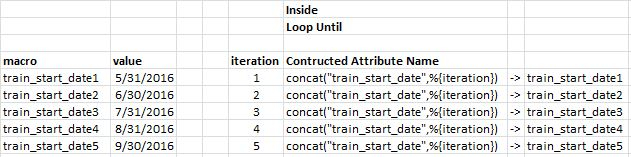Any help appreciated! Thanks.

-Noel
Tagged:

• 12Posts: 2,717Community Manager
Solution Accepted
hi @Noel ok been scratching my head for 5 min trying to figure out what the problem is. 😀Is this what you're looking for?

```<?xml version="1.0" encoding="UTF-8"?><process version="9.4.001">
<context>
<input/>
<output/>
<macros/>
</context>
<operator activated="true" class="process" compatibility="9.4.000" expanded="true" name="Process">
<parameter key="logverbosity" value="init"/>
<parameter key="random_seed" value="2001"/>
<parameter key="send_mail" value="never"/>
<parameter key="process_duration_for_mail" value="30"/>
<parameter key="encoding" value="SYSTEM"/>
<process expanded="true">
<parameter key="excel_file" value="/Users/genzerconsulting/Desktop/DateConstructionTest.v2.xlsx"/>
<parameter key="sheet_selection" value="sheet number"/>
<parameter key="sheet_number" value="1"/>
<parameter key="imported_cell_range" value="A1"/>
<parameter key="encoding" value="SYSTEM"/>
<parameter key="first_row_as_names" value="true"/>
<list key="annotations"/>
<parameter key="date_format" value=""/>
<parameter key="time_zone" value="SYSTEM"/>
<parameter key="locale" value="English (United States)"/>
<list key="data_set_meta_data_information">
<parameter key="0" value="macro.true.polynominal.attribute"/>
<parameter key="1" value="value.true.date.attribute"/>
</list>
<parameter key="datamanagement" value="double_array"/>
<parameter key="data_management" value="auto"/>
</operator>
<operator activated="true" class="concurrency:loop" compatibility="9.4.001" expanded="true" height="82" name="Loop" width="90" x="179" y="34">
<parameter key="number_of_iterations" value="5"/>
<parameter key="iteration_macro" value="iteration"/>
<parameter key="reuse_results" value="false"/>
<parameter key="enable_parallel_execution" value="false"/>
<process expanded="true">
<operator activated="true" breakpoints="after" class="generate_macro" compatibility="9.4.001" expanded="true" height="82" name="Generate Macro (2)" width="90" x="45" y="34">
<list key="function_descriptions">
<parameter key="foo" value="concat(&quot;train_start_date&quot;,%{iteration})"/>
</list>
</operator>
<operator activated="true" breakpoints="after" class="extract_macro" compatibility="9.4.001" expanded="true" height="68" name="Extract Macro (3)" width="90" x="179" y="34">
<parameter key="macro" value="%{foo}"/>
<parameter key="macro_type" value="data_value"/>
<parameter key="statistics" value="average"/>
<parameter key="attribute_name" value="value"/>
<parameter key="example_index" value="%{iteration}"/>
</operator>
<connect from_port="input 1" to_op="Generate Macro (2)" to_port="through 1"/>
<connect from_op="Generate Macro (2)" from_port="through 1" to_op="Extract Macro (3)" to_port="example set"/>
<portSpacing port="source_input 1" spacing="0"/>
<portSpacing port="source_input 2" spacing="0"/>
<portSpacing port="sink_output 1" spacing="0"/>
<portSpacing port="sink_output 2" spacing="0"/>
</process>
</operator>
<connect from_op="Read Excel" from_port="output" to_op="Loop" to_port="input 1"/>
<connect from_op="Loop" from_port="output 1" to_port="result 1"/>
<portSpacing port="source_input 1" spacing="0"/>
<portSpacing port="sink_result 1" spacing="0"/>
<portSpacing port="sink_result 2" spacing="0"/>
</process>
</operator>
</process>
```

Scott

• Member Posts: 437Unicorn
One way would be to loop your examples, then extract macro to store your Excell fields as macro and then generate macro to alter these values for your final macro value.

Or you could generate a new attribute using your Excell data and then extract this new attribute using the extract macro operator using a loop.
• Member Posts: 81Maven
Hi @kayman -

Thanks for your response. Did you have a chance to look at the process I uploaded, because I’m not sure what the difference is between what you suggested and what I was trying to do.

The crux of the matter is that I want to be absolutely sure the macro values agree with the number of the iteration running. That’s why I was trying to concatenate the iteration number onto a string. I can’t figure out how to reference the value of the macro whose name is the same as the resulting string...
• Member Posts: 437Unicorn
Ouch, I responded from my tablet in my lazy chair, so not able to check your stuff. At least we think alike :-)

Looks like Scott is more active and has a solution but if not I definitely will have a closer look at it.
• Member Posts: 81Maven
No worries! I may have misunderstood what you wrote; I barely know up from down in this domain.
• Member Posts: 81Maven
• Member Posts: 81Maven
• Member Posts: 81Maven
Hi Scott / @sgenzer

The specs for my request were probably wrong-headed. I.E. I was probably going about the task the wrong way.

I tried using the macros and their values from your example in Filter Examples (process and input files attached). I'm wondering if that operator doesn't take macros for inputs or if I'm doing it wrong.

To back up and give you some context, my desire to create a configurable, iterative training and testing process. I want to train a model on some span of time (that is passed as a parameter) and then test on another variable span of time (also passed as an input).The process would step forward by the amount of the test span and repeat the process.

I probably did a terrible job explaining this. Let me know if I can provide further clarification.
• Member Posts: 81Maven
Scott / @sgenzer -

"Filter Example Range" seems to support macros, so I'll work with that. It'll also sidestep the date format problem I'm having.

• Member Posts: 81Maven
Scott / @sgenzer -

How do I go about requesting that the "Filter Example" operator be enhanced to support macros?

As I said above, I can work with the ...Range flavor, but that involves me converting dates to period numbers and it is just a matter of time until I make a mistake.

Thanks,
Noel
• 12Administrator, Moderator, Employee, RapidMiner Certified Analyst, Community Manager, Member, University Professor, PM Moderator Posts: 2,717Community Manager
hi @Noel my apologies I think I dropped this thread. Thanks for pinging me again.

You can use macros for Filter Examples - particularly if you use the "expression" parameter rather than the "custom filters".

I'm a bit tangled on what you want to do now...can you re-clarify?Scott
• Member Posts: 81Maven
No worries!

I'm doing iterative training and testing... given some contiguous dates (say a week), train for some period of time before the target week,  then test on the target week, and repeat. (I couldn't figure out how to deal with the macros and syntax/formatting of dates, so I used period numbers.)

I have a fair amount of data and didn't want to manually run each week, so, in addition to my "real data", I input a table that looks like this:

interation_number, train_start_period, train_end_period, test_start_period, test_end_period

I put a row in the above table for each week I wanted to "run" and iterating over those rows did the repetitive training and testing programmaticallly (I wrote the output for each week to the database).

I'm doing bound-to-fail conversions from dates (as I logically think about my data set) to period numbers in order to make my process work. It's just a matter of time before I mess something up and I won't catch the mistake for a while because a range of numbers means nothing to me visually:

Optically, this is meaningful:

5, 9/30/17, 9/30/19, 10/1/19, 10/4/19

This, on the other hand, is not: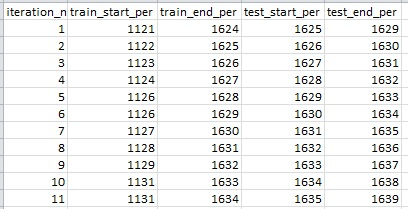Thanks!
-Noel

• 12Administrator, Moderator, Employee, RapidMiner Certified Analyst, Community Manager, Member, University Professor, PM Moderator Posts: 2,717Community Manager
ok @Noel had some time to tackle this this morning. Does this help?

```<?xml version="1.0" encoding="UTF-8"?><process version="9.5.000-BETA3">
<context>
<input/>
<output/>
<macros/>
</context>
<operator activated="true" class="process" compatibility="9.4.000" expanded="true" name="Process">
<parameter key="logverbosity" value="init"/>
<parameter key="random_seed" value="2001"/>
<parameter key="send_mail" value="never"/>
<parameter key="process_duration_for_mail" value="30"/>
<parameter key="encoding" value="SYSTEM"/>
<process expanded="true">
<parameter key="excel_file" value="DateConstructionTest.v2.xlsx"/>
<parameter key="sheet_selection" value="sheet number"/>
<parameter key="sheet_number" value="1"/>
<parameter key="imported_cell_range" value="A1"/>
<parameter key="encoding" value="SYSTEM"/>
<parameter key="first_row_as_names" value="true"/>
<list key="annotations"/>
<parameter key="date_format" value="MM/dd/yyyy"/>
<parameter key="time_zone" value="SYSTEM"/>
<parameter key="locale" value="English (United States)"/>
<list key="data_set_meta_data_information">
<parameter key="0" value="macro.true.polynominal.attribute"/>
<parameter key="1" value="value.true.date.attribute"/>
</list>
<parameter key="datamanagement" value="double_array"/>
<parameter key="data_management" value="auto"/>
</operator>
<operator activated="true" class="read_excel" compatibility="9.5.000-BETA3" expanded="true" height="68" name="Read Excel (2)" width="90" x="45" y="136">
<parameter key="excel_file" value="help.xlsx"/>
<parameter key="sheet_selection" value="sheet number"/>
<parameter key="sheet_number" value="1"/>
<parameter key="imported_cell_range" value="A1:S10485776"/>
<parameter key="encoding" value="SYSTEM"/>
<parameter key="first_row_as_names" value="true"/>
<list key="annotations"/>
<parameter key="date_format" value=""/>
<parameter key="time_zone" value="SYSTEM"/>
<parameter key="locale" value="English (United States)"/>
<list key="data_set_meta_data_information">
<parameter key="0" value="date.true.date.attribute"/>
<parameter key="1" value="attr1.true.real.attribute"/>
<parameter key="2" value="attr2.true.real.attribute"/>
<parameter key="3" value="attr3.true.real.attribute"/>
<parameter key="4" value="attr4.true.real.attribute"/>
<parameter key="5" value="attr5.true.real.attribute"/>
<parameter key="6" value="attr6.true.real.attribute"/>
<parameter key="7" value="attr7.true.integer.attribute"/>
<parameter key="8" value="attr8.true.integer.attribute"/>
<parameter key="9" value="attr9.true.integer.attribute"/>
<parameter key="10" value="attr10.true.integer.attribute"/>
<parameter key="11" value="attr11.true.integer.attribute"/>
<parameter key="12" value="attr12.true.real.attribute"/>
<parameter key="13" value="attr13.true.real.attribute"/>
<parameter key="14" value="attr14.true.real.attribute"/>
<parameter key="15" value="focus_attribute.true.real.attribute"/>
<parameter key="16" value="focus_attr_dod_diff.true.real.attribute"/>
<parameter key="17" value="focus_attr_20per_stdev.true.real.attribute"/>
<parameter key="18" value="label.true.polynominal.attribute"/>
</list>
<parameter key="datamanagement" value="double_array"/>
<parameter key="data_management" value="auto"/>
</operator>
<operator activated="true" class="concurrency:loop" compatibility="9.5.000-BETA3" expanded="true" height="103" name="Loop" width="90" x="246" y="34">
<parameter key="number_of_iterations" value="4"/>
<parameter key="iteration_macro" value="iteration"/>
<parameter key="reuse_results" value="false"/>
<parameter key="enable_parallel_execution" value="false"/>
<process expanded="true">
<operator activated="true" class="extract_macro" compatibility="9.5.000-BETA3" expanded="true" height="68" name="Extract Macro" width="90" x="45" y="34">
<parameter key="macro" value="startDate"/>
<parameter key="macro_type" value="data_value"/>
<parameter key="statistics" value="average"/>
<parameter key="attribute_name" value="value"/>
<parameter key="example_index" value="%{iteration}"/>
<description align="center" color="transparent" colored="false" width="126">startDate</description>
</operator>
<operator activated="true" class="generate_macro" compatibility="9.5.000-BETA3" expanded="true" height="82" name="Generate Macro" width="90" x="179" y="34">
<list key="function_descriptions">
<parameter key="iterationPlusOne" value="1+eval(%{iteration})"/>
</list>
<description align="center" color="transparent" colored="false" width="126">iterationPlusOne</description>
</operator>
<operator activated="true" class="extract_macro" compatibility="9.5.000-BETA3" expanded="true" height="68" name="Extract Macro (2)" width="90" x="313" y="34">
<parameter key="macro" value="endDate"/>
<parameter key="macro_type" value="data_value"/>
<parameter key="statistics" value="average"/>
<parameter key="attribute_name" value="value"/>
<parameter key="example_index" value="%{iterationPlusOne}"/>
<description align="center" color="transparent" colored="false" width="126">endDate</description>
</operator>
<operator activated="true" class="generate_macro" compatibility="9.5.000-BETA3" expanded="true" height="82" name="Generate Macro (3)" width="90" x="447" y="34">
<list key="function_descriptions">
<parameter key="startDate" value="date_str_custom(date_parse_custom(%{startDate},&quot;MMM dd, yyyy&quot;),&quot;MM/dd/yyyy&quot;)"/>
<parameter key="endDate" value="date_str_custom(date_parse_custom(%{endDate},&quot;MMM dd, yyyy&quot;),&quot;MM/dd/yyyy&quot;)"/>
</list>
<description align="center" color="transparent" colored="false" width="126">startDate&lt;br/&gt;endDate</description>
</operator>
<operator activated="true" breakpoints="after" class="filter_examples" compatibility="9.5.000-BETA3" expanded="true" height="103" name="Filter Examples" width="90" x="447" y="238">
<parameter key="parameter_expression" value=""/>
<parameter key="condition_class" value="custom_filters"/>
<parameter key="invert_filter" value="false"/>
<list key="filters_list">
<parameter key="filters_entry_key" value="date.ge.%{startDate}"/>
<parameter key="filters_entry_key" value="date.lt.%{endDate}"/>
</list>
<parameter key="filters_logic_and" value="true"/>
</operator>
<connect from_port="input 1" to_op="Extract Macro" to_port="example set"/>
<connect from_port="input 2" to_op="Filter Examples" to_port="example set input"/>
<connect from_op="Extract Macro" from_port="example set" to_op="Generate Macro" to_port="through 1"/>
<connect from_op="Generate Macro" from_port="through 1" to_op="Extract Macro (2)" to_port="example set"/>
<connect from_op="Extract Macro (2)" from_port="example set" to_op="Generate Macro (3)" to_port="through 1"/>
<connect from_op="Filter Examples" from_port="example set output" to_port="output 1"/>
<portSpacing port="source_input 1" spacing="0"/>
<portSpacing port="source_input 2" spacing="147"/>
<portSpacing port="source_input 3" spacing="0"/>
<portSpacing port="sink_output 1" spacing="0"/>
<portSpacing port="sink_output 2" spacing="0"/>
</process>
</operator>
<connect from_op="Read Excel" from_port="output" to_op="Loop" to_port="input 1"/>
<connect from_op="Read Excel (2)" from_port="output" to_op="Loop" to_port="input 2"/>
<connect from_op="Loop" from_port="output 1" to_port="result 1"/>
<portSpacing port="source_input 1" spacing="0"/>
<portSpacing port="sink_result 1" spacing="0"/>
<portSpacing port="sink_result 2" spacing="0"/>
</process>
</operator>
</process>
```

• Member Posts: 81Maven
Hi Scott-

Thank you. This looks very promising. I haven't tried it out yet, but this seems to check all the boxes.

I'll be back to you.
• Member Posts: 81Maven
Hi Scott / @sgenzer -

I'm getting this error (in my process):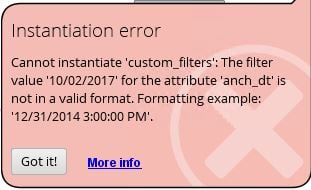I think I did everything as you did in the example process: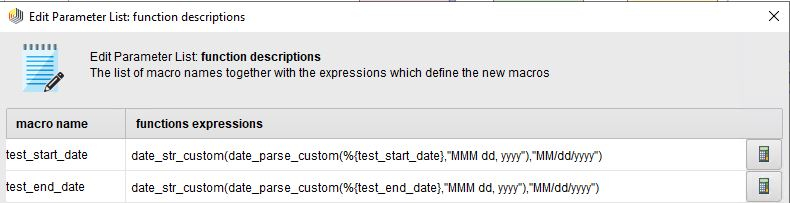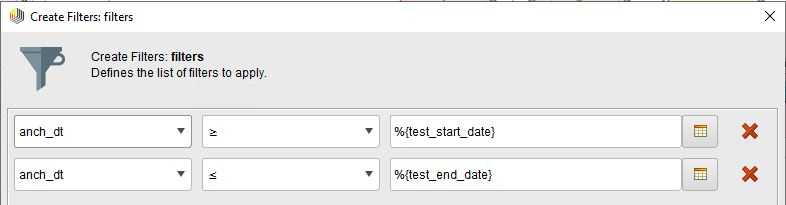As you know, the version you sent me works: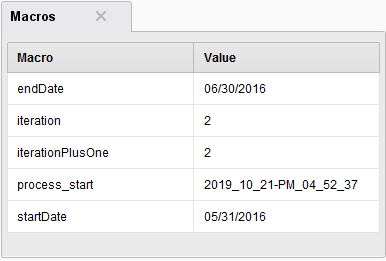Any idea why the "MM/dd/yyyy" format sometimes works and sometimes doesn't?
• 12Administrator, Moderator, Employee, RapidMiner Certified Analyst, Community Manager, Member, University Professor, PM Moderator Posts: 2,717Community Manager
hi @Noel dates & times are weird. No surprise that sometimes things are not parsed properly. You could try using Trim - maybe you have leading or trailing blank spaces? Otherwise I'd have to look at the data set.
• Member Posts: 81Maven
Grrr.

I tried: date_str_custom(date_parse_custom(trim(%{test_start_date}),"MMM dd, yyyy"),"MM/dd/yyyy") in my process to no avail.

I then added my current input file (and syntax) to your process and it works fine (attached).

Is there some way I can send you my process directly? email?